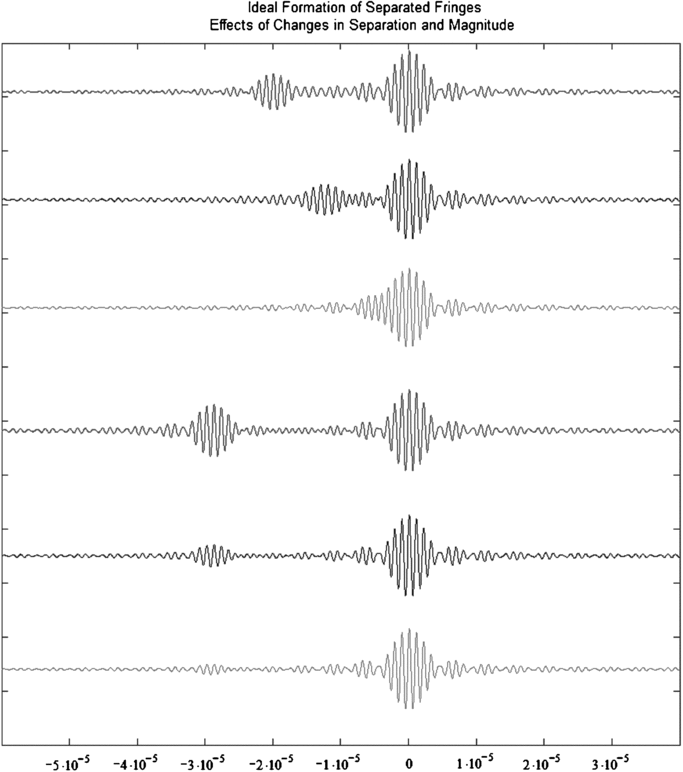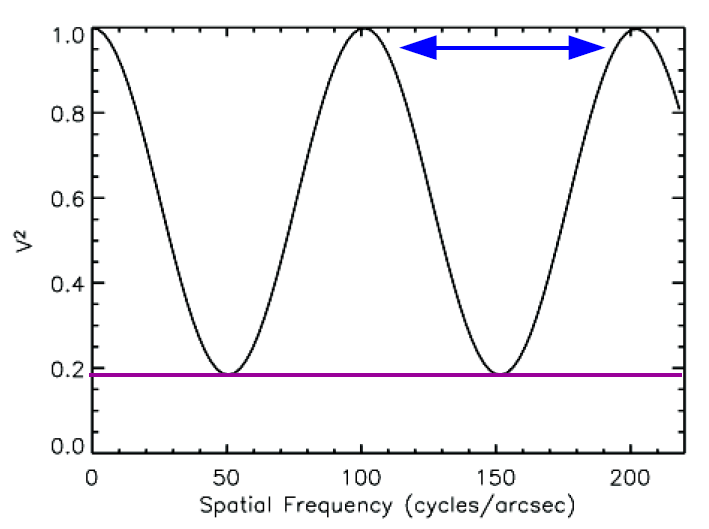# Companion Detection Limits

There are two ways that a binary star can be observed using an interferometer.  For binaries wider than the coherence length of the beam combiner, the binary will appear as a "Separated Fringe Packet" where a fringe is detected for each binary component.  The separation between the fringe packets gives the binary separation.  For a binary where the separation is smaller than the coherence length, then the two fringe packets from each component will overlap and a modulation can be measured in the visibilities and/or closure phases from the combined fringe packet.  The table below gives the separation range and magnitude sensitivities for binary companions resolvable using different beam combiners at the CHARA Array.

 Combiner Method Magnitude Difference Range of Separations* References CLASSIC/CLIMB Separated Fringe Packets ΔK < 1.5 mag 8 - 80 mas Farrington et al. (2010); Raghavan et al. (2012) CLASSIC Visibility modulation ΔK < 3 mag 0.5 - 8 mas Schaefer et al. (2018) CLIMB Visibility and closure phase modulation ΔK < 2 mag 0.5 - 8 mas MIRC Visibility and closure phase modulation ΔH < 6 mag 0.5 - 54 mas Gallenne et al. (2015) PAVO Visibility modulation ΔR < 3 mag 0.2 - 50 mas * The Range of Separations is based on the longest 331 m baseline assuming the wavelength band identified in the Magnitude Difference column.  These separation ranges will scale by a factor of 331.0/B where B is the length of the baseline being used.

### Separated Fringe Packet Binaries

Separated Fringe Packet binaries can be detected by beam combiners like CLASSIC and CLIMB that scan through the fringe window. The minimum separation for this technique is set by the coherence length of the beam combiner given by λ2/Δλ, where λ is the central wavelength and Δλ is the width of the bandpass filter.  The maximum separation is set by the length of the dither scan.

• For CLASSIC/CLIMB in the K-band (λ = 2.13 μm, Δλ = 0.3489 μm), the coherence length is 13.0 μm.

• For CLASSIC/CLIMB in the H-band (λ = 1.6731 μm, Δλ = 0.2854 μm), the coherence length is 9.8 μm.

• For CLASSIC, the short-scan length is 90 μm, medium-scan length is 120 μm, and long-scan length is 150 μm.

The separation in microns [ρ(μm)] can be converted to a separation in milli-arcsec [ρ(mas)] using the formula: ρ(mas) = 206.265 ρ(μm)/B(m) where B(m) is the baseline length in meters.  For the longest 331 m baseline at the CHARA Array using the longest dither scan, separated fringe packets can be measured with separations between 8-80 mas in the K-band.  Companions can be detected down to a magnitude difference of ΔK < 1.5 mag (Raghavan et al. 2012).Examples of separated fringe packet binaries from Farrington et al. (2010).  The top three scans show the effects of changing the binary separation at a fixed magnitude difference of Δm = 1 mag.  The bottom three scans show the effect of changing the magnitude difference (Δm = 0.5, 1.5, and 2.5 mag) at a fixed separation.  The separation between the two fringes gives the projected separation of the binary along the baseline while the magnitude difference determines the ratio of the fringe amplitudes.

### Modulated Fringe Packet Binaries

For binaries with overlapping fringe packets, the minimum separation is set by the resolution of the interferometer and is given by 0.5λ/B, where λ is the wavelength of light and B is the baseline length.  The resolution limit for the longest 331 m baseline at the CHARA Array is 0.20 mas in the R-band, 0.52 mas in the H-band, and 0.66 mas in the K-band.  The maximum separation that can be resolved is set by the coherence length of the beam combiner given by λ2/Δλ, where λ is the central wavelength and Δλ is the width of the bandpass filter.

• For CLASSIC/CLIMB in the K-band (λ = 2.13 μm, Δλ = 0.3489 μm), the coherence length is 13.0 μm.

• For CLASSIC/CLIMB in the H-band (λ = 1.6731 μm, Δλ = 0.2854 μm), the coherence length is 9.8 μm.

• For MIRC in the H-band (central λ = 1.62 μm, Δλ = 0.03 μm for the width of the 8 spectral channels), the coherence length is 86 μm.

• For PAVO in the R-band (median λ = 716 nm, Δλ = 6.4 nm for the width of a spectral channel), the coherence length is 80 μm.

The separation in microns [ρ(μm)] can be converted to a separation in milli-arcsec [ρ(mas)] using the formula: ρ(mas) = 206.265 ρ(μm)/B(m) where B(m) is the baseline length in meters. The table at the top of the page lists the range of separations (in mas) that can be resolved by each beam combiner using the longest 331 m baseline

The faintest companion that can be detected using the modulated fringe packet technique can be estimated as follows:

• For MIRC, Gallenne et al. (2015) determined a dynamic range of 1:200 (ΔH < 6 mag) for binaries with separations smaller than 50 mas.  The precision closure phases measured with MIRC (Zhao et al. 2011) provide good sensitivity to faint companions.

• For CLASSIC, Schaefer et al. (2018) estimated a maximum magnitude difference of ΔH < 2.9 mag based on a typical 4.5% scatter in the visibilities.

• For PAVO, an expected 5% precision on the calibrated visibilities would provide sensitivity down to ΔR = 2.7 mag.Example visibility curve for a binary star. The separation between the peaks in the visibility curve provides a measurement of the binary separation while the minimum visibility reflects the flux ratio between the components